Here is a function with the shape of a W:

t = chebfun(@(t) t);
f = 3*min(abs(t+.4),abs(t-.3));
ax = [-1.2 1.2 0 2.4];
plot(f), axis(ax), axis square, grid on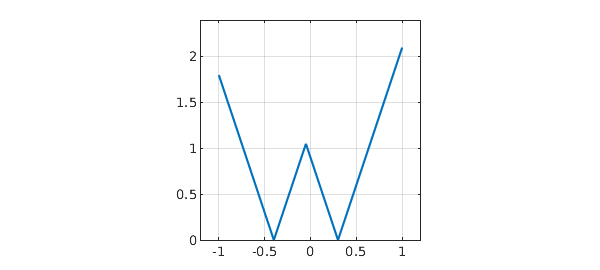And here is a narrow function with integral equal to $1$:

h = 0.1;
s = chebfun(@(s) s,[-h h]);
g = (h-abs(s))/h^2;
plot(g,'k'), axis([-1 1 0 12]), grid on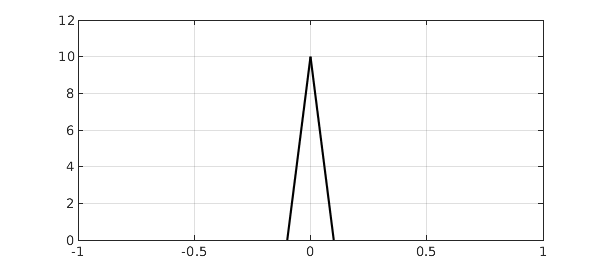If we convolve the two functions, we get a W with rounded corners. At the ends, the "rounding" has brought the values down to $0$:

f2 = conv(f,g);
plot(f2), axis(ax), axis square, grid on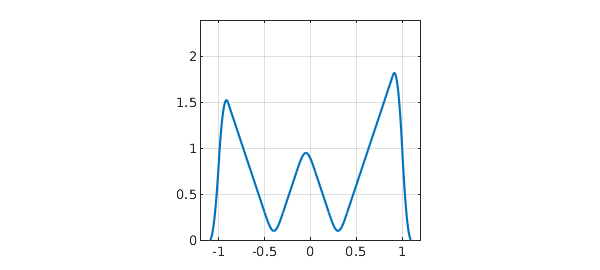Let's try a similar but different computation in which the W is not a real function of a real variable, but a complex function of a real parameter. Here is that complex function:

W = t + 1i*f(t);
plot(W,'r'), axis(ax), axis square, grid on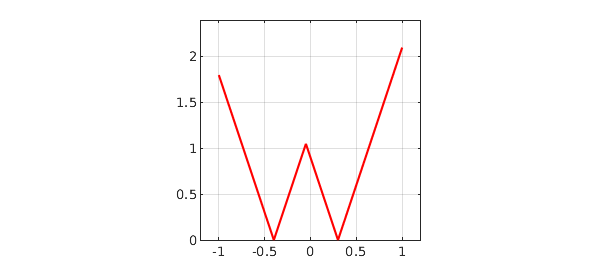And here is its convolution with g:

W2 = conv(W,g);
plot(W2,'r'), axis(ax), axis square, grid on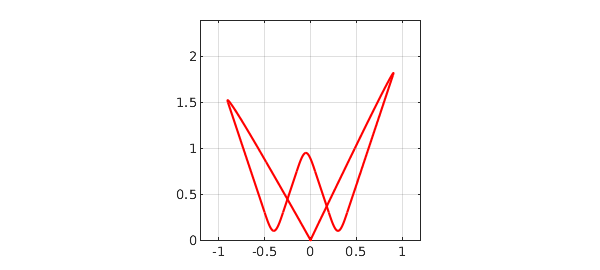Do you understand why this picture looks different from the previous one?